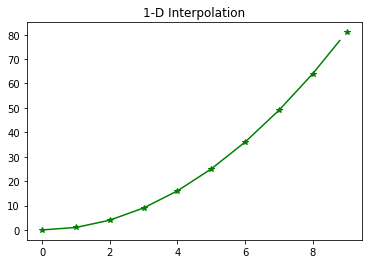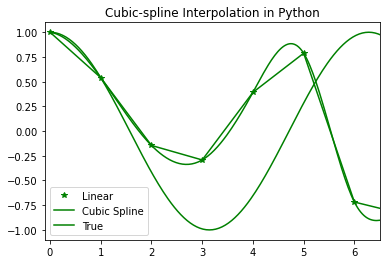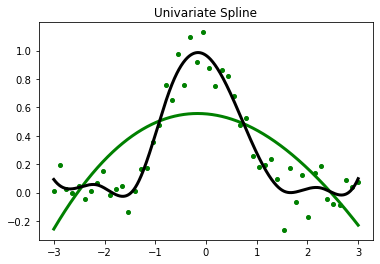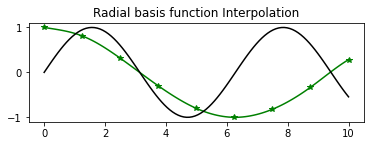Related Articles

# SciPy Interpolation

• Last Updated : 21 Apr, 2021

In this article, we will learn Interpolation using the SciPy module in Python. First, we will discuss interpolation and its types with implementation.

### Interpolation and Its Types

Interpolation is a technique of constructing data points between given data points. The scipy.interpolate is a module in Python SciPy consisting of classes, spline functions, and univariate and multivariate interpolation classes. Interpolation is done in many ways some of them are :

• 1-D Interpolation
• Spline Interpolation
• Univariate Spline Interpolation
• RBF Interpolation

Let’s discuss all the methods one by one and visualize the results.

### 1-D Interpolation

To create a function based on fixed data points, scipy.interpolate.interp1d is used. It takes data points x and y and returns a function that can be called with new x and returns the corresponding y point.

Syntax: scipy.interpolate.interp1d(x , y , kind , axis , copy , bounds_error , fill_value , assume_sorted)

## Python

 `# Import the required Python libraries``import` `matplotlib.pyplot as plt``from` `scipy ``import` `interpolate``import` `numpy as np`` ` `# Initialize input values x and y``x ``=` `np.arange(``0``, ``10``)``y ``=` `x``*``*``2`` ` `# Interpolation``temp ``=` `interpolate.interp1d(x, y)``xnew ``=` `np.arange(``0``, ``9``, ``0.2``)``ynew ``=` `temp(xnew)`` ` `plt.title(``"1-D Interpolation"``)``plt.plot(x, y, ``'*'``, xnew, ynew, ``'-'``, color``=``"green"``)``plt.show()`

Output:### Spline Interpolation

In spline interpolation, a spline representation of the curve is computed, and then the spline is computed at the desired points. The function splrep is used to find the spline representation of a curve in a two-dimensional plane.

• To find the B-spline representation of a 1-D curve, scipy.interpolate.splrep is used.

Syntax: scipy.interpolate.splrep(x, y, w, xb, xe, k, task, s, t, full_output, per, quiet)

• To compute a B-spline or its derivatives, scipy.interpolate.splev is used.

Syntax: scipy.interpolate.splev(x , tck , der , ext)

## Python

 `# Import the required Python libraries``import` `numpy as np``import` `matplotlib.pyplot as plt``from` `scipy ``import` `interpolate`` ` `# Initialize the input values``x ``=` `np.arange(``0``, ``10``)``y ``=` `np.cos(x``*``*``3``)`` ` `# Interpolation``# To find the spline representation of a ``# curve in a 2-D plane using the function ``# splrep``temp ``=` `interpolate.splrep(x, y, s``=``0``)``xnew ``=` `np.arange(``0``, np.pi``*``*``2``, np.pi``/``100``)``ynew ``=` `interpolate.splev(xnew, temp, der``=``0``)`` ` `plt.figure()`` ` `plt.plot(x, y, ``'*'``, xnew, ynew, xnew, np.cos(xnew),``         ``x, y, ``'b'``, color``=``"green"``)`` ` `plt.legend([``'Linear'``, ``'Cubic Spline'``, ``'True'``])``plt.axis([``-``0.1``, ``6.5``, ``-``1.1``, ``1.1``])``plt.title(``'Cubic-spline Interpolation in Python'``)``plt.show()`

Output:### Univariate Spline

It is a 1-D smoothing spline that fits a given group of data points. The scipy.interpolate.UnivariateSpline is used to fit a spline y = spl(x) of degree k to the provided x, y data. s specifies the number of knots by specifying a smoothing condition. The scipy.interpolate.UnivariateSpline. set_smoothing_factor: Spline computation with the given smoothing factor s and with the knots found at the last call.

Syntax: scipy.interpolate.UnivariateSpline( x, y, w, bbox, k, s, ext)

## Python

 `# Import the required libraries``import` `matplotlib.pyplot as plt``from` `scipy.interpolate ``import` `UnivariateSpline`` ` `x ``=` `np.linspace(``-``3``, ``3``, ``50``)``y ``=` `np.exp(``-``x``*``*``2``) ``+` `0.1` `*` `np.random.randn(``50``)``plt.title(``"Univariate Spline"``)``plt.plot(x, y, ``'g.'``, ms``=``8``)`` ` `# Using the default values for the ``# smoothing parameter``spl ``=` `UnivariateSpline(x, y)``xs ``=` `np.linspace(``-``3``, ``3``, ``1000``)``plt.plot(xs, spl(xs), ``'green'``, lw``=``3``)`` ` `# Manually change the amount of smoothing``spl.set_smoothing_factor(``0.5``)``plt.plot(xs, spl(xs), color``=``'black'``, lw``=``3``)``plt.show()`

Output:### Radial basis function for Interpolation

The scipy.interpolate.Rbf is used for interpolating scattered data in n-dimensions. The radial basis function is defined as corresponding to a fixed reference data point. The scipy.interpolate.Rbf is a class for radial basis function interpolation of functions from N-D scattered data to an M-D domain.

Syntax: scipy.interpolate.Rbf(*args)

## Python

 `# Import the required libraries``import` `numpy as np``from` `scipy.interpolate ``import` `Rbf``import` `matplotlib.pyplot as plt`` ` `# setup the data values``x ``=` `np.linspace(``0``, ``10``, ``9``)``y ``=` `np.cos(x``/``2``)``xi ``=` `np.linspace(``0``, ``10``, ``110``)`` ` `# Interpolation using RBF``rbf ``=` `Rbf(x, y)``fi ``=` `rbf(xi)`` ` `plt.subplot(``2``, ``1``, ``2``)``plt.plot(x, y, ``'*'``, color``=``"green"``)``plt.plot(xi, fi, ``'green'``)``plt.plot(xi, np.sin(xi), ``'black'``)``plt.title(``'Radial basis function Interpolation'``)``plt.show()`

Output:Attention geek! Strengthen your foundations with the Python Programming Foundation Course and learn the basics.

To begin with, your interview preparations Enhance your Data Structures concepts with the Python DS Course. And to begin with your Machine Learning Journey, join the Machine Learning – Basic Level Course

My Personal Notes arrow_drop_up